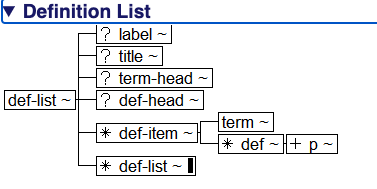#### Hierarchy Diagrams

Hierarchy diagrams are graphical representations of the structure of elements, for elements that have complex structure. An element with a simple model, such as an “or” list (that is, where any of a number of elements may occur inside it in any order), for example Paragraph (<p>), is not illustrated with a hierarchy diagram. An element that must have one of these, followed by any of this list of things, followed be either this or that, will be illustrated with a hierarchy diagram.

All of the Hierarchy diagrams may be reached through the Getting Started Section. An individual diagram for a specific element can be reached through the Related Resources subsection on the element page:

Figure 27. Finding the hierarchy diagrams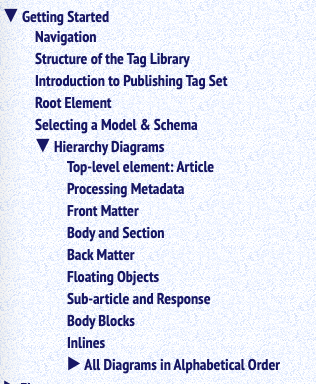The first page of the Hierarchy Diagrams section describes the symbols used in the diagrams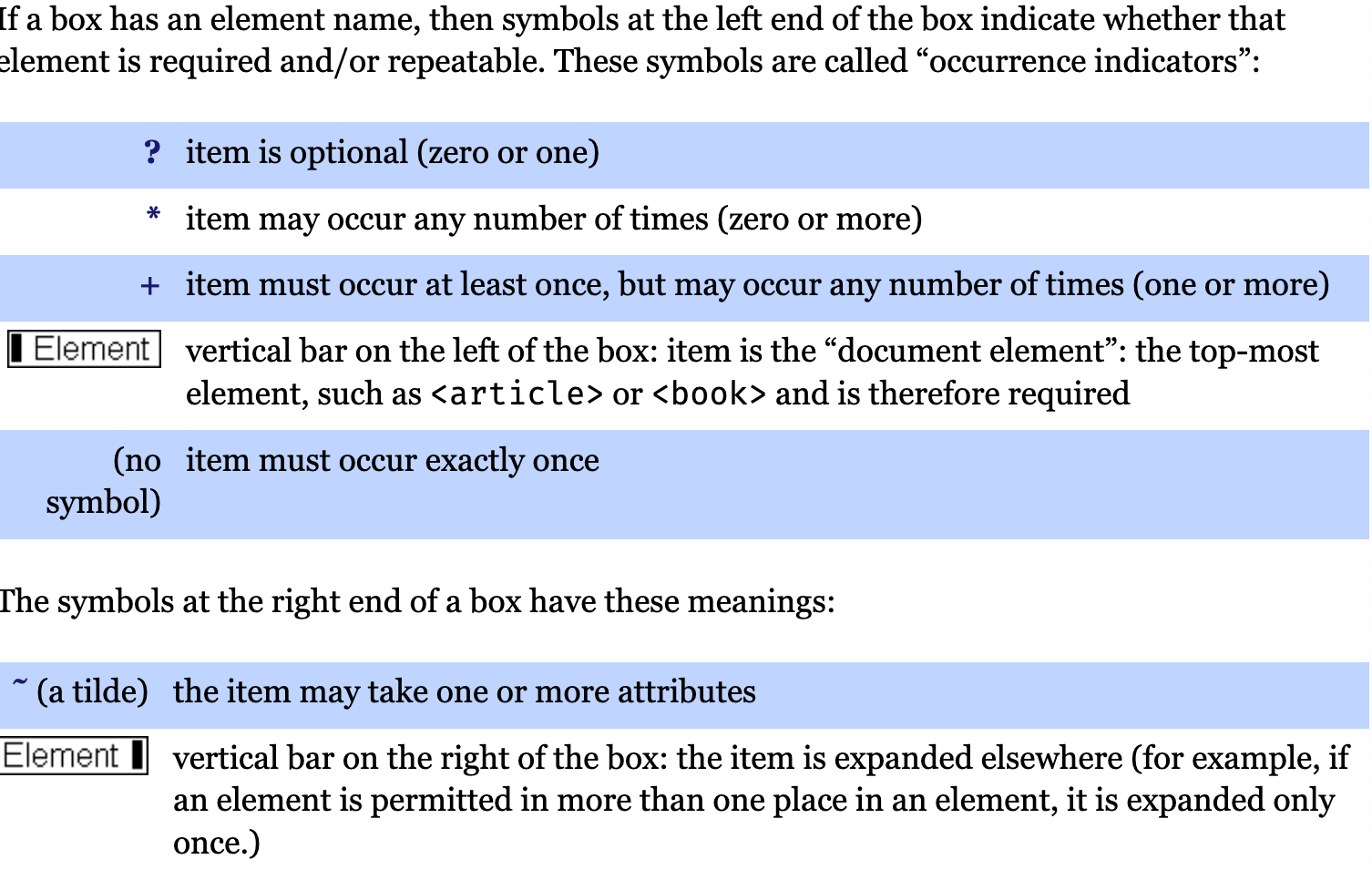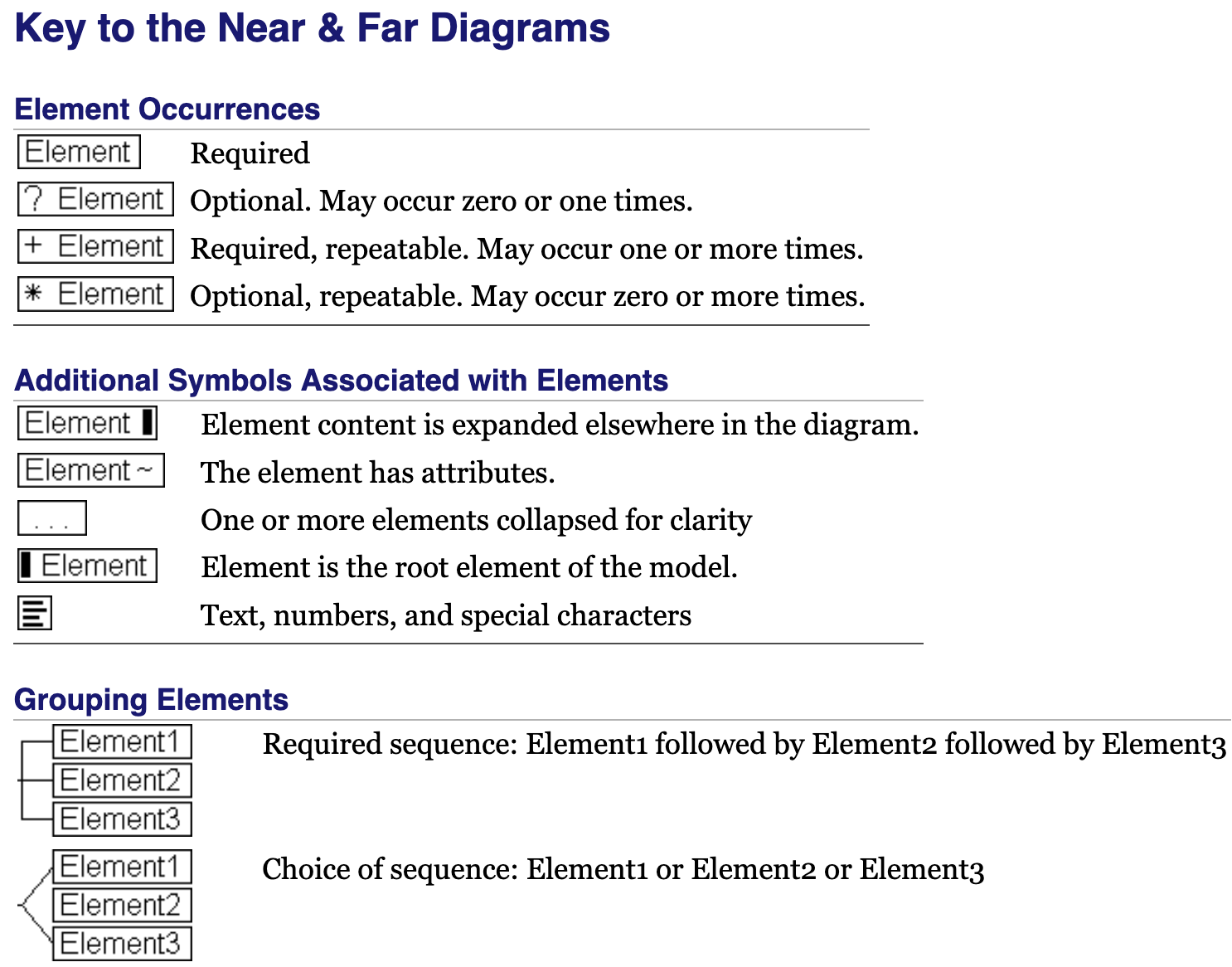Figure 28. The hierarchy diagram for Article (<article>)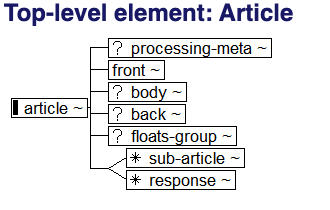Figure 29. The hierarchy diagram for Back Matter (<back>)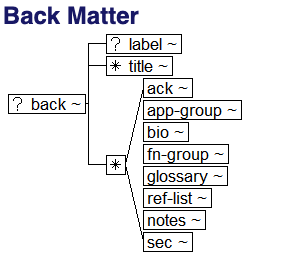Figure 30. The hierarchy diagram for Definition List (<def-list>)